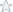# University of Phoenix (Axia) - MAT117 Appendix E Solutions

Axia College Material

Appendix E

Application Practice

Answer the following questions. Use Equation Editor to write mathematical expressions and equations. First, save this file to your hard drive by selecting Save As from the File menu. Click the white space below each question to maintain proper formatting.

Hint for this assignment: Pay attention to the units of measure. You may have to convert from feet to miles several times in this assignment. You can use 1 mile = 5,280 feet for your conversions.

1. Many people know that the weight of an object varies on different planets, but did you know that the weight of an object on earth also varies according to the elevation of the object? In particular, the weight of an object follows this equation:, where C is a constant, and r is the distance that the object is from the center of the earth.

a. Solve the equation for r.

b. Suppose that an object is 100 pounds when it is at sea level. Find the value of C that makes the equation true. (Sea level is 3,963 miles from the center of the earth.)
,

c. Use the value of C you found in the previous question to determine how much the object would weigh in

i. Death Valley (282 feet below sea level)

ii. The top of Mt McKinley (20,320 feet above sea level)

2. The equation gives the distance, D, in miles that a person can see to the horizon from a height, h, in feet.

a. Solve this equation for h.

b. Long’s Peak in the Rocky Mountain National Park, is 14,255 feet in elevation. How far can you see to the horizon from the top of Long’s Peak? Can you see Cheyenne, Wyoming (about 89 miles away)? Explain your answer.

Solution are created using MathType (as a Microsoft Word Document) and received a grade of 95%.

Subjects: Solving Linear Equations involving Radicals
Level: College / University
Tags:

### Axia, University of Phoenix, Appendix E, Radicals

Price\$4.95

Math Genius
 Frederick Burke Member Since: March 2003 Customer Rating: 94.7% Projects Completed: 795 Total Earnings: *Private* +1 Ratings from clients: 115
Project Details
Subjects: Solving Linear Equations involving Radicals
Level: College / University
Tags:

## Axia, University of Phoenix, Appendix E, Radicals

Customer Reviews
Rated 7 Times
Rating( 4.5 / 5 Stars)

Reviews of the solutions left by other users
No customer has left a detailed review for these math solutions. Be the first one to purchase them and leave a review for others!

Not exactly what you are looking for?
We regularly update our math homework solutions library and are continually in the process of adding more samples and complete homework solution sets. If you do not find what you are looking for, just go ahead and place an order for a custom created homework solution. You can hire/pay a math genius to do your homework for you exactly to your specifications.